Question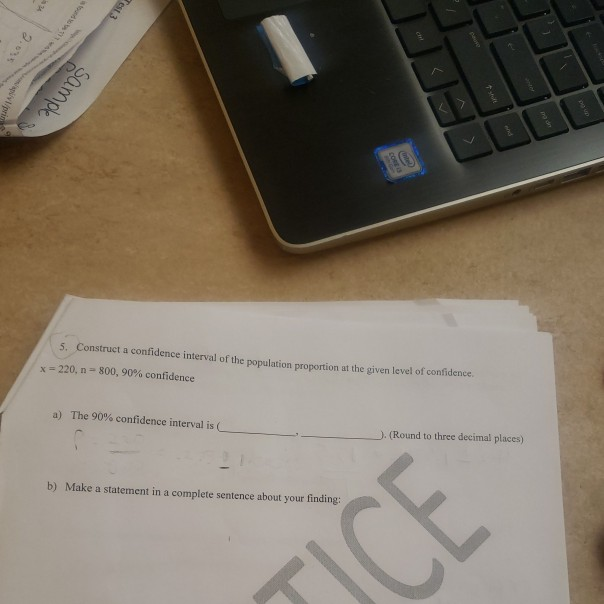number 5 A & B. please explain.

a)

Solution :

Given that,

n = 800

x = 220

Point estimate = sample proportion =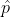= x / n = 0.275

1 -= 1 - 0.275 = 0.725

At 90% confidence level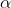= 1 - 90%= 1 - 0.90 =0.10/2 = 0.05

Z/2 = Z0.05 = 1.645 ( Using z table )

Margin of error = E = Z/ 2 *((* (1 -)) / n)

= 1.645 (( 0.275( 0.725 ) / 800 )

= 0.026

A 90% confidence interval for population proportion p is ,- E < p <+ E

0.275 - 0.026 < p < 0.275 + 0.026

0.249 < p < 0.301

(0.249,0.301)

b)

A 90% confidence interval for the population proportion p (0.249,0.301) is fall between lower bound and upper bound

#### Earn Coins

Coins can be redeemed for fabulous gifts.

Similar Homework Help Questions
• ### Construct a confidence interval of the population proportion at the given level of confidence. x- 120,...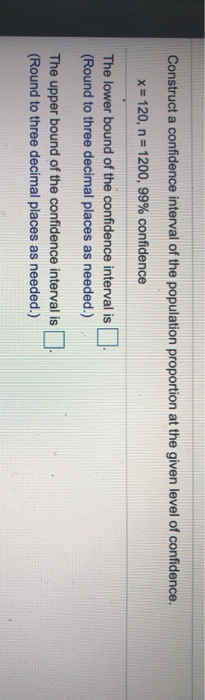Construct a confidence interval of the population proportion at the given level of confidence. x- 120, n 1200, 99% confidence The lower bound of the confidence interval is (Round to three decimal places as needed.) The upper bound of the confidence interval is (Round to three decimal places as needed.) Construct a 99% confidence interval of the population proportion using the given information. X 105, n 150 The lower bound is The upper bound is (Round to three decimal places...

• ### Construct a 90​% confidence interval to estimate the population proportion with a sample proportion equal to...

Construct a 90​% confidence interval to estimate the population proportion with a sample proportion equal to 0.44 and a sample size equal to 100. A 90​% confidence interval estimates that the population proportion is between a lower limit of blank and an upper limit of. ​(Round to three decimal places as​ needed.)

• ### Construct a confidence interval of the population proportion at the given level of confidence. x =...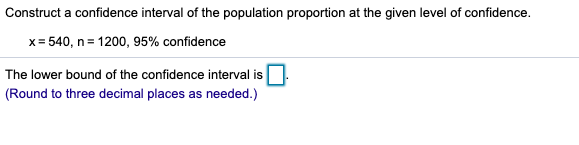Construct a confidence interval of the population proportion at the given level of confidence. x = 540, n= 1200, 95% confidence The lower bound of the confidence interval is (Round to three decimal places as needed.)

• ### Construct a confident interval of the population proportion at the given level of confidence. X=120 n=300...Construct a confident interval of the population proportion at the given level of confidence. X=120 n=300 90% Confidence Thanks! 8 of 9 (0 Construct a confidence interval of the population proportion at the given level of confidence xz 120, n: 300, 90% confidence The lower bound is The upper bound is (Round to three decimal places as needed) Enter your answer in each of the answer boxes. O Type here to search

• ### Construct a confidence interval of the population proportion at the given level of confidence x=860, n=1100,...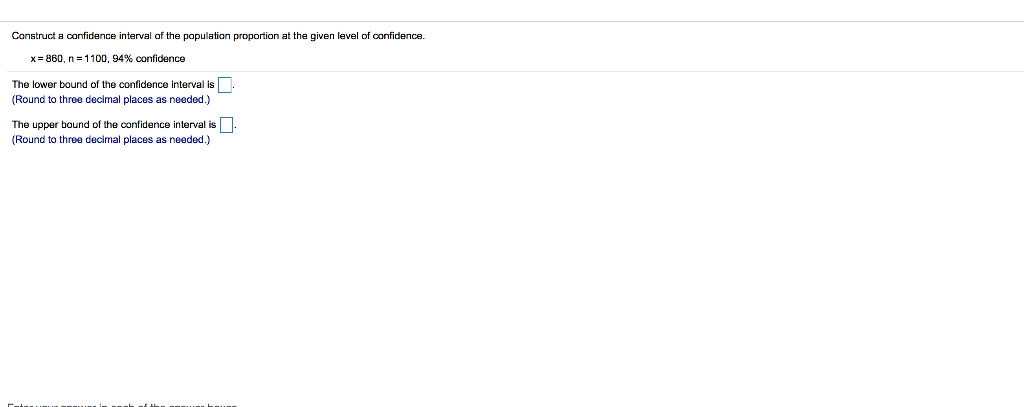Construct a confidence interval of the population proportion at the given level of confidence x=860, n=1100, 94% confidence The lower bound of the confidence interval is (Round to three decimal places as needed.) The upper bound of the confidence interval is (Round to three decimal places as needed.)

• ### Use the given data to construct a confidence interval for the population proportion p of the...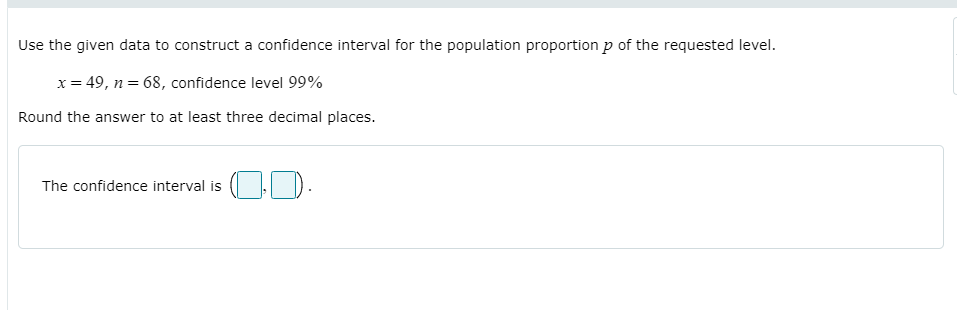Use the given data to construct a confidence interval for the population proportion p of the requested level. x= 49, n= 68, confidence level 99% Round the answer to at least three decimal places. The confidence interval is

• ### 9.1.19 Construct a confidence interval of the population proportion at the given level of confidence. x=540,...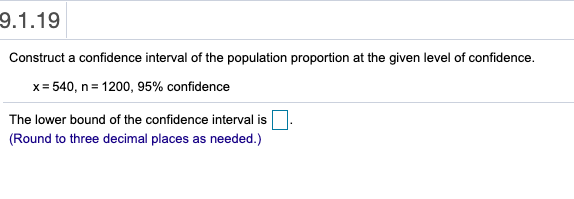9.1.19 Construct a confidence interval of the population proportion at the given level of confidence. x=540, n= 1200, 95% confidence . The lower bound of the confidence interval is (Round to three decimal places as needed.)

• ### Construct a confidence interval of the population proportion at the given level of confidence. x =...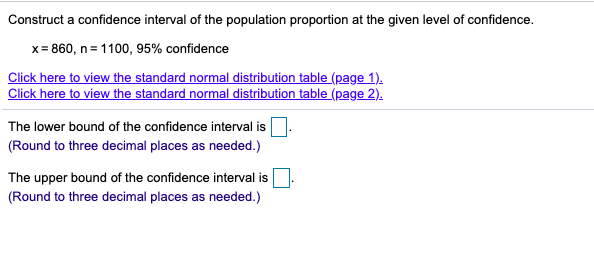Construct a confidence interval of the population proportion at the given level of confidence. x = 860, n= 1100, 95% confidence Click here to view the standard normal distribution table (page 1). Click here to view the standard normal distribution table (page 2). The lower bound of the confidence interval is (Round to three decimal places as needed.) The upper bound of the confidence interval is (Round to three decimal places as needed.)

• ### Use the given data to construct a confidence interval for the population proportion p of the...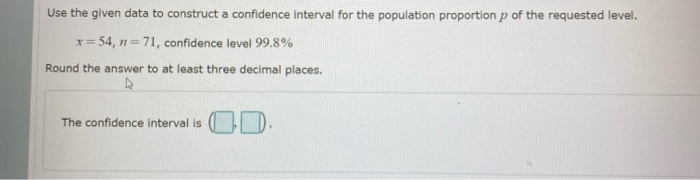Use the given data to construct a confidence interval for the population proportion p of the requested level. x=54, n=71, confidence level 99.8% Round the answer to at least three decimal places. The confidence interval is C D .

• ### Construct a confidence interval of the population proportion at the given level of confidence x= 40,...Construct a confidence interval of the population proportion at the given level of confidence x= 40, n= 200, 99% confidence The 99% confidence interval is (Use ascending order. Round to three decimal places as needed.) Enter your answer in each of the answer boxes scs100 theme4.p.ppt O Type here to searc